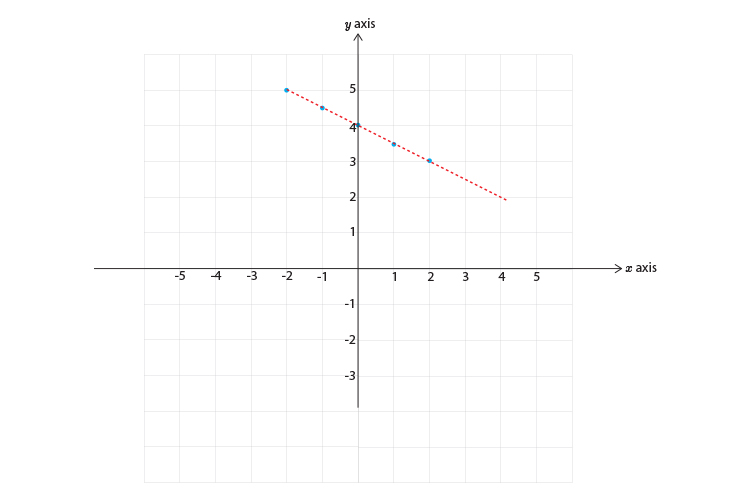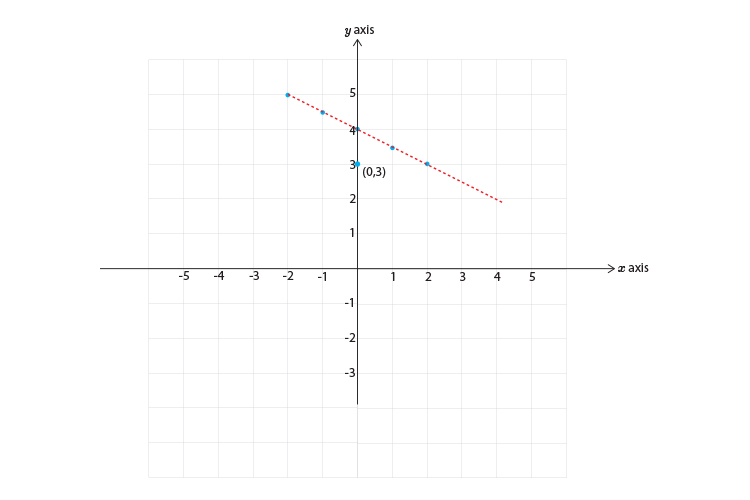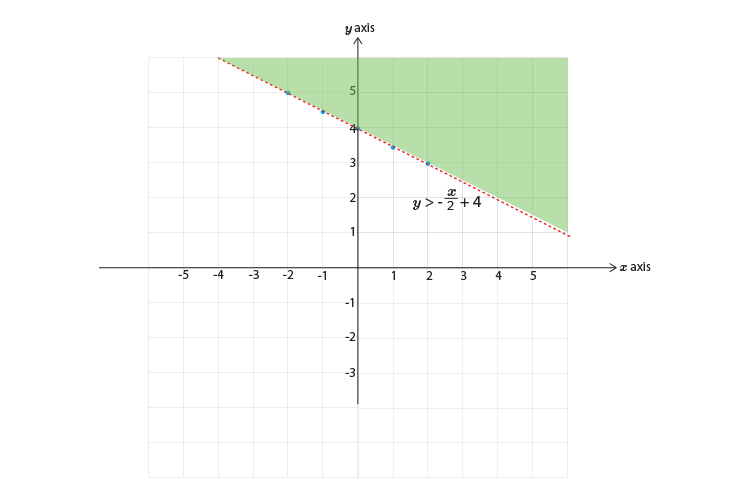# Inequalities and graphs example 1

Graph the following inequality.

x+2y>8

Subtract x  from both sides to get y  on its own.

x-x+2y>8-x

2y>8-x

Divide both sides by 2 to get y  on its own.

(2y)/2>8/2-x/2

y>8/2-x/2

y>4-x/2

y>\-x/2+4

First, let's calculate some points on the graph.

 x=-2 then y>\-(-2/2)+4=5 x=-1 then y>\-(-1/2)+4=4.5 x=0 then y>\-(0/2)+4=4 x=1 then y>\-(1/2)+4=3.5 x=2 then y>\-(2/2)+4=3

Now draw a graph and mark on the above points and don't forget to use just a dotted line.Now pick a point NOT on the line:Choose x=0  and y=3

y>4-x/2

and 3>4-0/2

3>4

This reads 3 is greater than 4 which is of course incorrect.

So this DOES NOT SATISFY THE INEQUALITY.